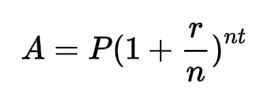top of page

# How Savings Grow - the Rule of 72A plant seed needs fertile ground, light, water, and time to grow. Your money needs a stable interest rate, reinvested interests (i.e. compound interest), and time. So similarly to a plant, you care for it providing the nutrition, and wait until the harvesting date. You don't take anything out from your investment in between the planting and the harvesting.

You have decided to start saving towards a target. But how does your money grow, how long does it take until you can "harvest" your growth?

Exact answer: use the compound interest formula (and a calculator)

Maybe you started to look up the answer and came across the term of compound interest and the formula on how to calculate the money growth:Where A = final amount

P = initial balance of the principal

r = interest rate

n = number of times the interest was applied per time period

t = number of time period elapsed

So to get an idea of how much your saved money will be worth after several years, you either take your calculator or find an online version and run the numbers.

Simple and quick estimate: the rule of 72:

Already the ancient civilizations of the Greeks, Romans, and Mesopotamians have used the interest concept. Back then the main use was within agricultural and land transactions, but also for money loans. Already during those days the lenders and the borrows needed to know how much an investment will be worth over time. So they used a simplified approach for getting a quick understanding.

The first official reference for the Rule of 72 is in the book "Summa de arithmetica" (Venice, 1494. Fol. 181, n. 44) of Luca Pacioli (1445–1514). He presents the rule in a discussion regarding the estimation of the doubling time of an investment, but does not derive or explain the rule, and it is thus assumed that the rule predates Pacioli by some time. He wrote (roughly translated):

"In wanting to know of any capital, at a given yearly percentage, in how many years it will double adding the interest to the capital, keep as a rule [the number] 72 in mind, which you will always divide by the interest, and what results, in that many years it will be doubled. Example: When the interest is 6 percent per year, I say that one divides 72 by 6; 12 results, and in 12 years the capital will be doubled."

Applied to your savings the rule of 72 says:

The estimated number of years for doubling your initial investment = 72 / annual compound interest rate.
Note that this is only an estimate. It assumes the interest is compounded monthly and works best for interest rates of 3-15%.

Let's make an example:

You invest 10'000\$ with a 6% interest rate. After 72/6 = 12 years there will be 20'000\$ in your account. After a total of 24 years, the money will be worth 40'000\$.

More efficient saving method: Add money to your savings regularly, then the impact is even bigger.

The picture below shows three different scenarios, all using a 6% annual savings rate :

1. Start with 10'000\$ and wait for 45 years -> total money invested 10'000\$So even without a big initial investment sum at hand, the gain you can make over time, adding a smaller amount at a regular interval is remarkable. In our example, the money gained with interest over 45 years is:

1. 137'800\$

2. 328'500\$

3. 1'077'400\$

Do you want to explore more scenarios? Use an online calculator .

"How Savings Grow" will be the topic of our March zoom-meeting of the "Money Talk with a Daily Money Manager"-series hosted by Rita Kuehnis, SDS Smart Daily Services LLC. The meeting is scheduled for Thursday, March 18th at 6 - 7 pm PST. Register here